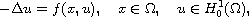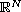Electron. J. Differential Equations, Vol. 2016 (2016), No. 317, pp. 1-9.

### Sign-changing solutions for asymptotically linear Schrodinger equation in bounded domains Sitong Chen, Yinbin Li, Xianhua Tang

Abstract:whereis a bounded domain inand f(x,u) is asymptotically linear at infinity with respect to u. Inspired by the works of Salvatore  on sign-changing solutions, in whichis asymptotically linear at zero with respect to u, we prove, via the constraint variational method and the quantitative deformation lemma, that the equation possesses one sign-changing solution with exactly two nodal domains.Sitong Chen School of Mathematics and Statistics Central South University Changsha, 410083 Hunan, China email: mathsitongchen@163.com Yinbin Li School of Mathematics and Statistics Central South University Changsha, 410083 Hunan, China email: liyinbin1991@163.com Xianhua Tang School of Mathematics and Statistics Central South University Changsha, 410083 Hunan, China email: tangxh@mail.csu.edu.cn